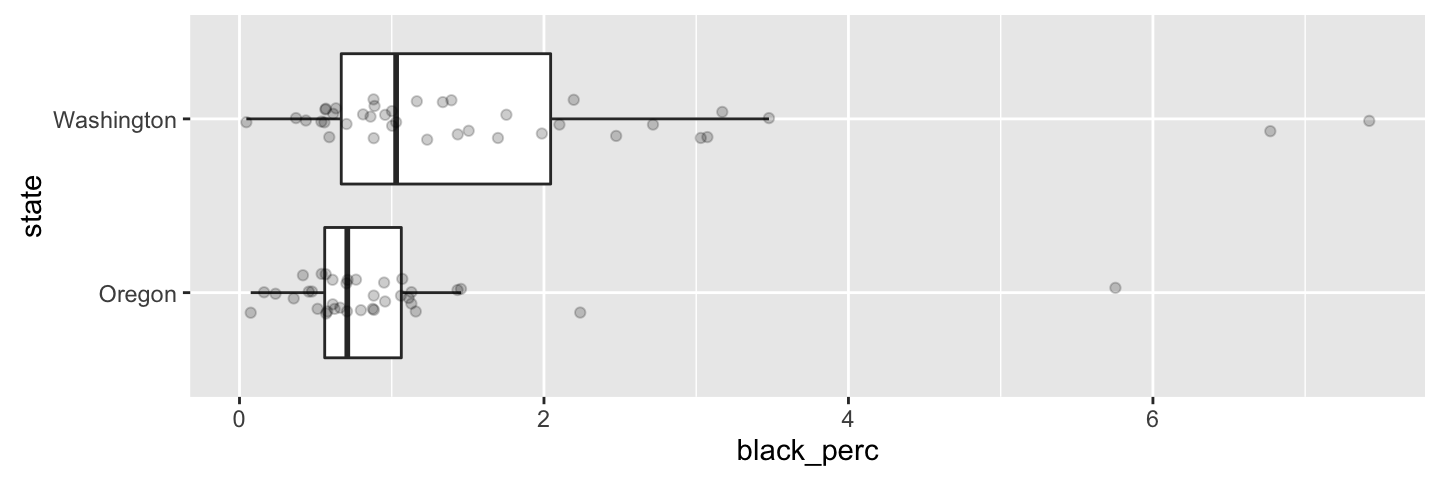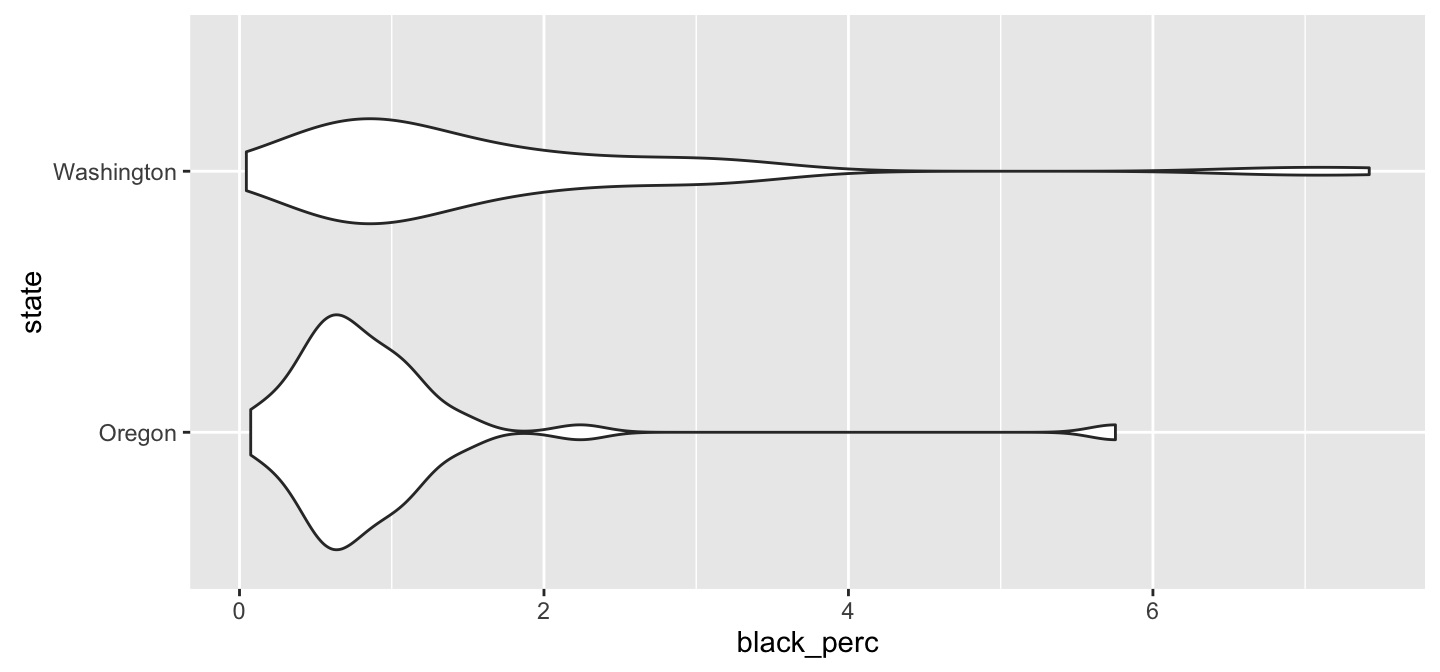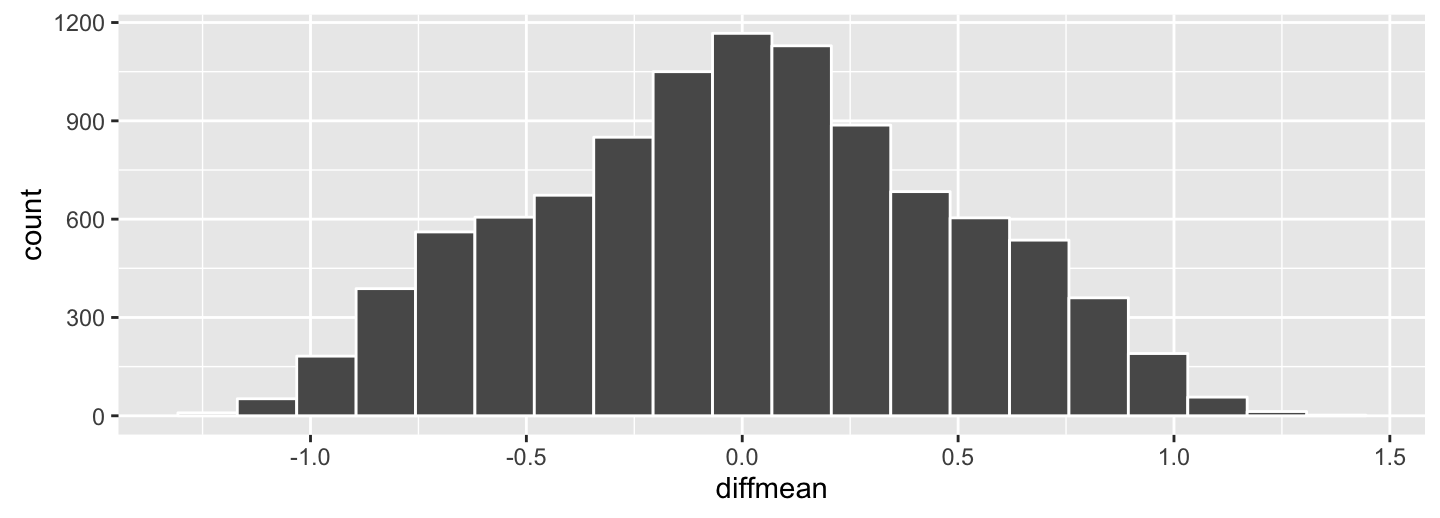## Annual County Resident Population Estimates by Age, Sex, Race, and Hispanic Origin

• Recall that from our hypothesis test from last time that we had evidence that the mean percentage of black residents by county was different for the states of Oregon and Washington.
• Your assignment was to look to see what the actual mean percentage of black residents was in our population:
library(readr)
cc_full <- read_csv("/shared/isma5720@pacificu.edu/cc_full.csv")

## Outlining the problem solution

• Lay out what you think the resulting data frame will look like
• What dplyr verbs are needed to accomplish this task?
• Write down the code via pencil-and-paper and then write the code in RStudio.

## Calculating the population means ($$\mu_{wa}$$ and $$\mu_{or}$$)

library(dplyr)
cc_full %>% filter(state %in% c("Washington", "Oregon")) %>%
group_by(state) %>%
summarize(mu = mean(black_perc))
## # A tibble: 2 × 2
##        state     mu
##        <chr>  <dbl>
## 1     Oregon 0.9226
## 2 Washington 1.6375
• So, as we found from our hypothesis test, we had reason to believe that Washington had a higher mean percentage of black residents per county than Oregon.
• Is the difference $$\mu_{wa} - \mu_{or}$$ practically different?

## Relationship between state and black_perc

Another important step is to visualize how the response variable changes based on the levels of the explanatory variable:

library(ggplot2); cc_full %>% filter(state %in% c("Washington", "Oregon")) %>%
ggplot(mapping = aes(x = state, y = black_perc)) +
geom_boxplot(outlier.shape = NA) +
geom_jitter(alpha = 0.2, height = 0, width = 0.3) +
coord_flip()## Relationship between state and black_perc

cc_full %>% filter(state %in% c("Washington", "Oregon")) %>%
ggplot(mapping = aes(x = state, y = black_perc)) +
geom_violin() +
coord_flip()• Hypothesis testing is usually a good start, but you may have asked "We have a statistically significant difference in the sample mean black percentage in Washington versus Oregon, but how much larger do we expect the Washington mean to be?"
• Confidence intervals help us to provide guidance to solve this problem.
• Recall the randomization distribution we created by generating many different differences in sample means via shuffling called rand_distn from last time. I've saved this into a R data file (rds) on the RStudio Server that we can name black_rand_distn in R:
black_rand_distn <- read_rds("/shared/isma5720@pacificu.edu/black_rand_distn.RDS")

## Plotting

black_rand_distn %>% ggplot(aes(x = diffmean)) +
geom_histogram(color = "white", bins = 20)• How could we extract the minimal value and maximum value from diffmean using dplyr?

## Getting the range

black_rand_distn %>% summarize(min_diff = min(diffmean),
max_diff = max(diffmean))
## # A tibble: 1 × 2
##   min_diff max_diff
##      <dbl>    <dbl>
## 1   -1.278    1.336
• Recall that this distribution assumes that the null hypothesis is true. We don't necessarily want that to be the case when providing a range of plausible values for $$\mu_{wa} - \mu_{or}$$.

• We are interested in the variability obtained via the black_rand_distn though.

• We'll see how to put this together next.

## Point estimate versus a range

Recall that we have $$\bar{x}_{wa} - \bar{x}_{or} = 0.9105771$$.

This is one guess. Why don't we just use that as our guess to what $$\mu_{wa} - \mu_{or}$$ is?

Point Estimate Confidence Interval## Confidence levels

• Usually what is done is to provide a confidence level corresponding to what percentage of middle values one would like to include in the range of plausible values for the population parameter.

• We want to incorporate the variability that is seen in looking at a variety of different samples, while also using our point estimate as the center of our confidence interval.

• So if we wanted a 95% confidence interval for $$\mu_{wa} - \mu_{or}$$, we could look at the 2.5 percentile/quantile and the 97.5 percentile/quantile of a shifted randomization distribution.

• Note that we used a 5% significance level on our hypothesis test. The results for our confidence interval will match up since the hypothesis tests looks out the outer 5% and the confidence intervals corresponds to the inner 95%.

## Creating the confidence interval

obs_diff <- 0.9105771
(shifted_black_rand <- black_rand_distn %>%
mutate(diffmean = obs_diff + diffmean) %>%
summarize(lower = quantile(diffmean, probs = 0.025),
upper = quantile(diffmean, probs = 0.975)))
## # A tibble: 1 × 2
##     lower upper
##     <dbl> <dbl>
## 1 0.02367 1.811

We are 95% confident that the true mean difference in the percentage of black residents by county in Washington versus Oregon is between 0.0237 and 1.8113 percent.

## Deciphering what this means

• In other words, we can expect the true mean percentage of black residents by county in Washington to be 0.0237% to 1.8113% higher than for Oregon.

• Since zero is not included in this confidence interval, we can conclude the same thing as we did for the hypothesis test: we have statistical significance between the two sample means at the 5% level.

• Recall that we calculated earlier that $$\mu_{wa} - \mu_{or} = 1.6375464 - 0.9225828 = 0.7149636$$.
• We can expect that if we had collected 100 different original samples and performed this same process on each of the different resulting shifted distributions, that 95 of them would have contained this true value of 0.7149636.

## The process for creating a confidence interval

• Obtain an original sample (at random) & compute the original sample statistic
• Lay out what the null and alternative hypotheses are
• Determine what sort of random process assumes $$H_0$$ while keeping true to how the original sample was selected and the original statistic was calculated
• Create the randomization distribution by repeating the process many times
• Shift this distribution by adding the original statistic (point estimate)
• Compute the appropriate percentiles from this shifted distribution
• 2.5 and 97.5 for 95% confidence level
• 5 and 95 for 90% confidence level
• Interpret the resulting confidence interval in the context of the problem

## Reviewing data for project

• I'd like to make sure your data file is read in to RStudio and that it is tidy
• As I go around the room, review your schedules in groups the week after Thanksgiving and determine times throughout the week when you are all available.

## To do for next time

• Enjoy the Thanksgiving break!